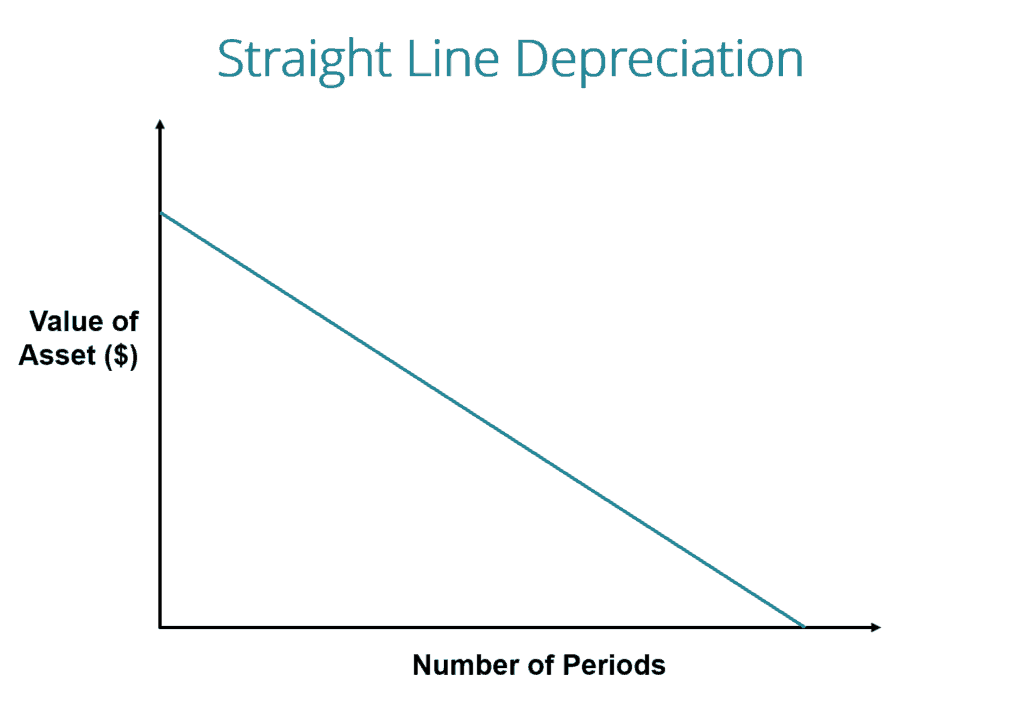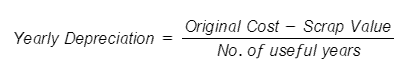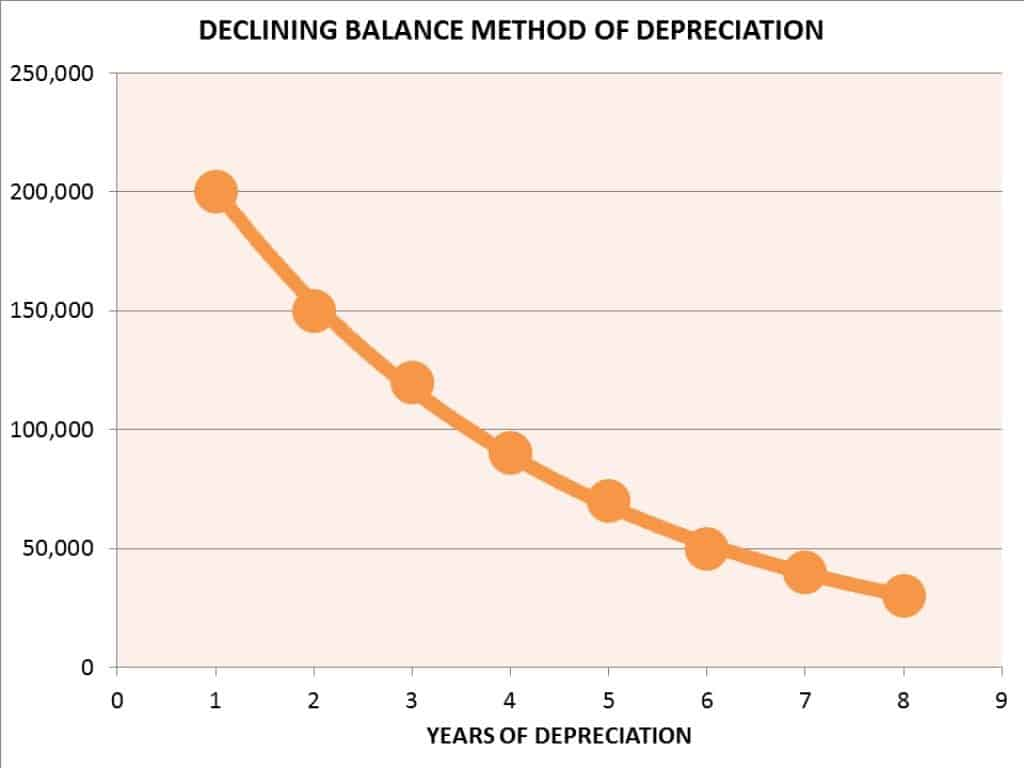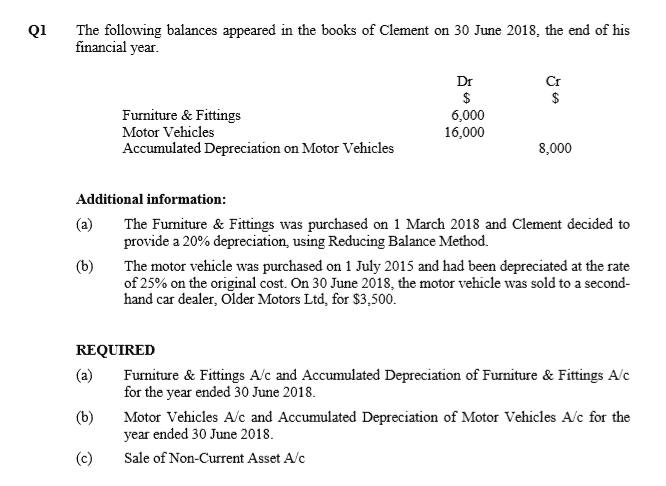Understanding Depreciation in Accounting ConceptDepreciation plays an important part in a business and can potentially be hard to calculate. When calculated wrongly or avoided as a whole, this can result in incurring losses for the business.

At your level, as students, this will no doubt lead to the deduction of marks in the exam. It is therefore important to understand the concept of depreciation and how it works. This is precisely what this article intends to do.

So first and foremost…

What exactly is depreciation?

To begin with, depreciation can be defined as a fall in the value of assets such as vehicles and machines. The important fact about depreciation to note here is that it is an allocation, rather than a valuation, process that dictates the cost of an asset over its useful life. Useful life typically refers to the estimated productive life of an asset.

What causes depreciation?

Here are 4 important causes of depreciation:

1) Wear and tear due to use or passage of time

As suggested by its heading, wear and tear come with 2 different aspects. The former refers to the gradual diminishing value of an asset as a result of its use in business operations to generate revenue. The latter refers to the physical deterioration of an asset as a result of exposure to natural elements such as the weather, wind, and rain. For example, a sofa in an office requires replacement such as excessive scratches and dirt.

2) Obsolescence

This is also a factor that results in the depreciation of non-current assets. It implies that an existing asset is becoming outdated due to the availability of a newer/better type of asset. This may arise from factors such as technological changes, improvement in production methods, change in market demand for the product or service output of the asset and legal reasons.

3) Depletion

The value of the non-current asset may decrease in value when the benefits of the non-current asset are consumed (used up) by the business. It usually applied to natural resources type of non-current assets.

4) Legal Rights

An asset may involve usage rights in which can only be used until a specific point in time. The owner will be required to give up ownership once its usage rights expire. The depreciation of such assets has to be done over time and not simply be written off upon expiration. Such assets may include patent rights or computer software.

How is depreciation calculated?

Today’s businesses use various methods to calculate depreciation on assets. For the purpose of the current POA syllabus, we will cover the 2 most common depreciation calculation methods. These are the Straight-line Depreciation and Reducing Balance depreciation methods.

Straight-line Depreciation MethodSource: Website

The straight-line depreciation method has an assumption that an asset depreciates at a constant rate over its useful life. This method spreads the cost of the non-current assets evenly over its useful life. Straight-line Depreciation Method is ideal for assets requiring negligible maintenance expenses and are not prone to technological obsolescence.

The straight-line depreciation method is calculated using the following formula:Or = (Original Cost – Scrap Value) x Depreciation Rate (%)

What are the advantages of using the Straight-line Depreciation Method?

• Easiest and the most straightforward method of depreciating an asset
• Does not require complex calculations, lesser margin of error
• The book value of an asset can be reduced to zero (if no scrap value)
• Since the asset is uniformly depreciated, it does not cause the variation in the Profit or loss due to depreciation expense while other depreciation methods can have an impact on income statement variations.

• Difficult to estimate the useful life of an asset
• Not justifiable because depreciation amount of an asset remains the same every year.

To have an understanding of straight-line depreciation method works, watch this video:

Reducing Balance Depreciation MethodSource: Website

Reducing-Balance depreciation method, also known as Diminishing-Balance method, refers to a constant rate of depreciation being applied to an asset’s book value each year, resulting in a faster rate of depreciation. There is then higher depreciation values in the early years of the life of an asset.

Reducing-Balance Depreciation Method will be useful for assets that require more repairs and maintenance expenses as time passes. This method is also appropriate for assets which are prone to technological obsolescence because it results in higher depreciation during the initial years of an asset’s life.

The reducing balance depreciation method is calculated using the following formula:

Yearly Depreciation = Net Book Value x Rate of Depreciation (%)

What are the advantages of using the Reducing-Balance Depreciation Method?

• Good method to record depreciation of assets that rapidly lose their value or become obsolete (e.g. a computer), hence, depicting fair market value on the balance sheet.
• Net income is reduced because of higher depreciation in the initial years. This leads to tax benefits due to lower tax outflows.

• Lower net income in the initial years of an asset as a result of the initial high rate of depreciation
• Not an ideal method for assets with a lower rate of depreciation such as office equipment

To have an understanding of how reducing balance depreciation method works, watch this video:

After looking at the theoretical aspect of depreciation, you might be asking…

.. How would this topic be tested in the examination?

For N-level (academic) – only non-current assets and depreciation would be tested.

For O-level – the non-current assets and depreciation would be tested together with the sale of non-current assets

Now that we have gone through the theoretical aspect of depreciation, we can move on to the practical aspect. Thus, we aim to provide you with a practical understanding of the depreciation and sale of non-current assets. Below is the guiding steps to approach a typical examination question on:

Non-current asset + Depreciation + Sale of non-current asset.

1) Transfer the cost of the sold non-current asset to Sale of Non-Current Asset a/c.

Dr Sale of Non-Current Asset a/c
Cr Non-Current Asset a/c

2) Transfer the accumulated depreciation of the sold non-current asset to Sale of Non-Current Asset a/c.

Dr Accumulated Depreciation of Non-Current Asset a/c
Cr Sale of Non-Current Asset a/c

3) Record the sales proceeds (sales price) of the sold non-current asset in the Sale of Non-Current Asset a/c.

Dr Cash at Bank / Cash in Hand / Other Receivable a/c
Cr Sale of Non-Current Asset a/c

4) Record the Profit / Loss of the sold non-current asset in the Sale of Non-Current Asset a/c.

Dr Profit and Loss a/c (Loss on Sale)
Cr Sale of Non-Current Asset a/c

Dr Sale of Non-Current Asset a/c
Cr Profit and Loss a/c (Profit on Sale)

Below is a worked example of a depreciation question done by one of our students.Depreciation is closely guided by an accounting concept called Matching Principle which stipulates the direction of a company to report an expense on its income statement in the period in which the related revenues are earned.

In dealing with depreciation and non-current assets, take note of the following key pointers.

For depreciation, you will have to read on the depreciation policy mentioned in the question. Identify the depreciation method needed to be calculated, whether using straight-line or reducing balance method. You are also required to identify whether there are any ‘special requirements’ stated in the question. For instance, will you be required to calculate a full year depreciation in the year of purchase and sale or using a pro-rate method according to months used.

Now, having explained these steps, scroll back up and have another look at the worked solution we have provided. See if you are able to understand why those answers are derived with the steps that we have elaborated earlier.

Conclusion

And that wraps up our explanation of the concept of depreciation! We hope this article has given you a better understanding of what depreciation is and how it works. This article may have clarified your theoretical doubt but in order to grasp its application well, you will have to practice consistently. If you still have further enquiries on the concept of depreciation and POA in general, feel free to contact us.

BEST POA provides dedicated POA Tuition Services. The lessons are conducted by Mr. Edmund Ling, an accounting tutor who has 28 years of tutoring experience and an illustrious professional career record.